Function Repository Resource:

# JacobiMatrix

Generate the Jacobi matrix corresponding to an orthogonal polynomial

Contributed by: Jan Mangaldan
 ResourceFunction["JacobiMatrix"][n,poly] yields the nth order Jacobi matrix corresponding to the orthogonal polynomial pn(x) represented by poly.

## Details and Options

A Jacobi matrix is a square tridiagonal matrix whose characteristic polynomial is a scalar multiple of the orthogonal polynomial pn(x).
The argument poly can be any of the following:
 "ChebyshevFirst" Chebyshev polynomial of the first kind ChebyshevT[n,x] "ChebyshevSecond" Chebyshev polynomial of the second kind ChebyshevU[n,x] "Hermite" Hermite polynomial HermiteH[n,x] "Laguerre" Laguerre polynomial LaguerreL[n,x] "Legendre" Legendre polynomial LegendreP[n,x] {"Gegenbauer",m} Gegenbauer polynomial GegenbauerC[n,m,z] {"Laguerre",a} associated Laguerre polynomial LaguerreL[n,a,x] {"Jacobi",a,b} Jacobi polynomial JacobiP[n,a,b,x]
Strings in specifications like "Legendre" and {"Jacobi",a,b} can be replaced with the corresponding built-in symbols, as in LegendreP and {JacobiP,a,b}.
The result of ResourceFunction["JacobiMatrix"] is a SparseArray.
ResourceFunction["JacobiMatrix"][n,poly,"Symmetric"True] generates a symmetric tridiagonal Jacobi matrix. ResourceFunction["JacobiMatrix"][n,poly,"Symmetric"False] generates an unsymmetric tridiagonal Jacobi matrix with 1's in the subdiagonal.

## Examples

### Basic Examples (1)

The Jacobi matrix corresponding to a Legendre polynomial:

 In:=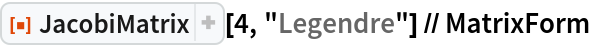Out=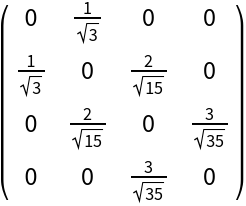### Scope (2)

Jacobi matrix of a Jacobi polynomial with symbolic parameters:

 In:=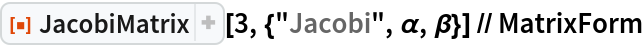Out=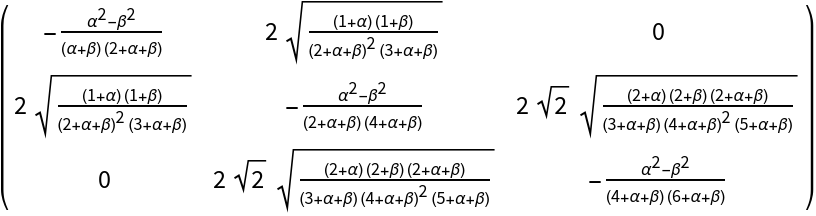An equivalent specification:

 In:=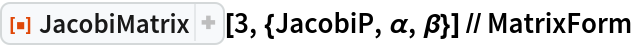Out=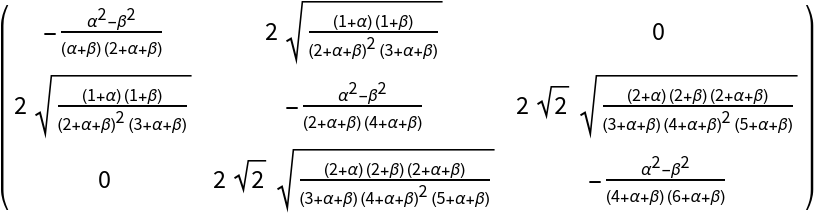### Options (1)

#### Symmetric (1)

Symmetric and unsymmetric Jacobi matrices for an associated Laguerre polynomial:

 In:=Out=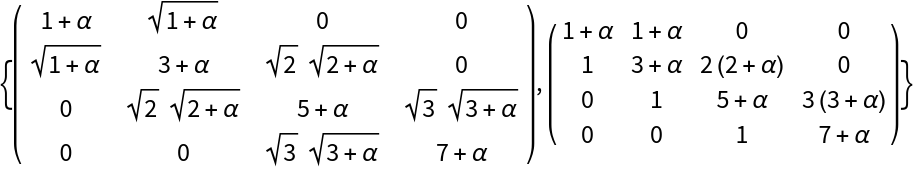### Applications (2)

Find the roots of an orthogonal polynomial by computing the eigenvalues of a Jacobi matrix:

 In:=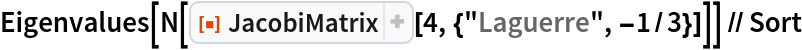Out=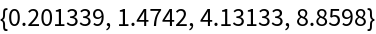Compare with the result of using NSolve:

 In:=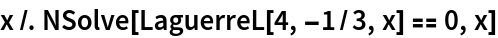Out=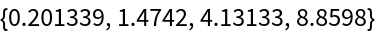### Properties and Relations (2)

The characteristic polynomial of a Jacobi matrix is a scalar multiple of the associated orthogonal polynomial:

 In:=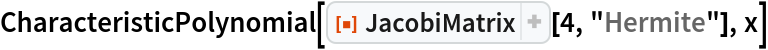Out=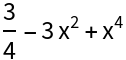In:=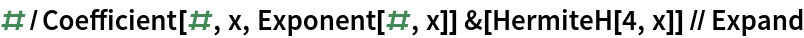Out=The unsymmetric and symmetric Jacobi matrices are related to each other through a similarity transformation:

 In:=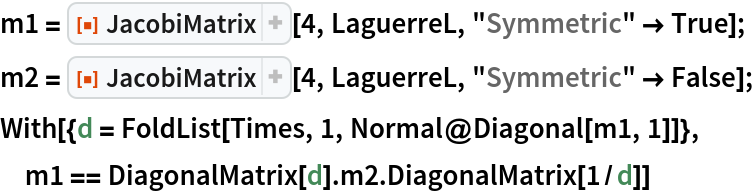Out=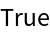## Version History

• 1.0.0 – 05 April 2021